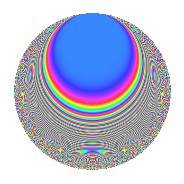# Properties

 Label 3311.1.hLevel 3311 Weight 1 Character orbit h Rep. character $$\chi_{3311}(3310,\cdot)$$ Character field $$\Q$$ Dimension 38 Newform subspaces 16 Sturm bound 352 Trace bound 7

# Related objects

## Defining parameters

 Level: $$N$$ = $$3311 = 7 \cdot 11 \cdot 43$$ Weight: $$k$$ = $$1$$ Character orbit: $$[\chi]$$ = 3311.h (of order $$2$$ and degree $$1$$) Character conductor: $$\operatorname{cond}(\chi)$$ = $$3311$$ Character field: $$\Q$$ Newform subspaces: $$16$$ Sturm bound: $$352$$ Trace bound: $$7$$

## Dimensions

The following table gives the dimensions of various subspaces of $$M_{1}(3311, [\chi])$$.

Total New Old
Modular forms 42 42 0
Cusp forms 38 38 0
Eisenstein series 4 4 0

The following table gives the dimensions of subspaces with specified projective image type.

$$D_n$$ $$A_4$$ $$S_4$$ $$A_5$$
Dimension 38 0 0 0

## Trace form

 $$38q + 30q^{4} + 26q^{9} + O(q^{10})$$ $$38q + 30q^{4} + 26q^{9} - 6q^{11} - 2q^{14} - 8q^{15} + 22q^{16} + 26q^{25} + 18q^{36} - 6q^{44} + 32q^{49} - 8q^{53} - 10q^{56} - 12q^{58} - 24q^{60} + 26q^{64} - 8q^{67} - 16q^{78} + 30q^{81} - 12q^{92} - 2q^{99} + O(q^{100})$$

## Decomposition of $$S_{1}^{\mathrm{new}}(3311, [\chi])$$ into newform subspaces

Label Dim. $$A$$ Field Image CM RM Traces $q$-expansion
$$a_2$$ $$a_3$$ $$a_5$$ $$a_7$$
3311.1.h.a $$1$$ $$1.652$$ $$\Q$$ $$D_{2}$$ $$\Q(\sqrt{-7})$$, $$\Q(\sqrt{-3311})$$ $$\Q(\sqrt{473})$$ $$-2$$ $$0$$ $$0$$ $$-1$$ $$q-2q^{2}+3q^{4}-q^{7}-4q^{8}-q^{9}-q^{11}+\cdots$$
3311.1.h.b $$1$$ $$1.652$$ $$\Q$$ $$D_{3}$$ $$\Q(\sqrt{-3311})$$ None $$-1$$ $$-1$$ $$-1$$ $$1$$ $$q-q^{2}-q^{3}-q^{5}+q^{6}+q^{7}+q^{8}+\cdots$$
3311.1.h.c $$1$$ $$1.652$$ $$\Q$$ $$D_{3}$$ $$\Q(\sqrt{-3311})$$ None $$-1$$ $$1$$ $$1$$ $$1$$ $$q-q^{2}+q^{3}+q^{5}-q^{6}+q^{7}+q^{8}+\cdots$$
3311.1.h.d $$1$$ $$1.652$$ $$\Q$$ $$D_{3}$$ $$\Q(\sqrt{-3311})$$ None $$1$$ $$-1$$ $$-1$$ $$-1$$ $$q+q^{2}-q^{3}-q^{5}-q^{6}-q^{7}-q^{8}+\cdots$$
3311.1.h.e $$1$$ $$1.652$$ $$\Q$$ $$D_{3}$$ $$\Q(\sqrt{-3311})$$ None $$1$$ $$1$$ $$1$$ $$-1$$ $$q+q^{2}+q^{3}+q^{5}+q^{6}-q^{7}-q^{8}+\cdots$$
3311.1.h.f $$1$$ $$1.652$$ $$\Q$$ $$D_{2}$$ $$\Q(\sqrt{-7})$$, $$\Q(\sqrt{-3311})$$ $$\Q(\sqrt{473})$$ $$2$$ $$0$$ $$0$$ $$1$$ $$q+2q^{2}+3q^{4}+q^{7}+4q^{8}-q^{9}-q^{11}+\cdots$$
3311.1.h.g $$2$$ $$1.652$$ $$\Q(\sqrt{-3})$$ $$D_{6}$$ None $$\Q(\sqrt{473})$$ $$-2$$ $$0$$ $$0$$ $$-1$$ $$q-q^{2}+\zeta_{6}^{2}q^{7}+q^{8}-q^{9}-q^{11}+\cdots$$
3311.1.h.h $$2$$ $$1.652$$ $$\Q(\sqrt{3})$$ $$D_{6}$$ $$\Q(\sqrt{-3311})$$ None $$-2$$ $$0$$ $$0$$ $$2$$ $$q-q^{2}-\beta q^{3}-\beta q^{5}+\beta q^{6}+q^{7}+q^{8}+\cdots$$
3311.1.h.i $$2$$ $$1.652$$ $$\Q(\sqrt{3})$$ $$D_{6}$$ $$\Q(\sqrt{-3311})$$ None $$2$$ $$0$$ $$0$$ $$-2$$ $$q+q^{2}-\beta q^{3}-\beta q^{5}-\beta q^{6}-q^{7}-q^{8}+\cdots$$
3311.1.h.j $$2$$ $$1.652$$ $$\Q(\sqrt{-3})$$ $$D_{6}$$ None $$\Q(\sqrt{473})$$ $$2$$ $$0$$ $$0$$ $$1$$ $$q+q^{2}-\zeta_{6}^{2}q^{7}-q^{8}-q^{9}-q^{11}+\cdots$$
3311.1.h.k $$3$$ $$1.652$$ $$\Q(\zeta_{18})^+$$ $$D_{9}$$ $$\Q(\sqrt{-3311})$$ None $$0$$ $$0$$ $$0$$ $$-3$$ $$q+(-\beta _{1}+\beta _{2})q^{2}+\beta _{1}q^{3}+(1-\beta _{1}+\cdots)q^{4}+\cdots$$
3311.1.h.l $$3$$ $$1.652$$ $$\Q(\zeta_{18})^+$$ $$D_{9}$$ $$\Q(\sqrt{-3311})$$ None $$0$$ $$0$$ $$0$$ $$3$$ $$q+(\beta _{1}-\beta _{2})q^{2}-\beta _{1}q^{3}+(1-\beta _{1})q^{4}+\cdots$$
3311.1.h.m $$3$$ $$1.652$$ $$\Q(\zeta_{18})^+$$ $$D_{9}$$ $$\Q(\sqrt{-3311})$$ None $$0$$ $$0$$ $$0$$ $$-3$$ $$q+(-\beta _{1}+\beta _{2})q^{2}-\beta _{1}q^{3}+(1-\beta _{1}+\cdots)q^{4}+\cdots$$
3311.1.h.n $$3$$ $$1.652$$ $$\Q(\zeta_{18})^+$$ $$D_{9}$$ $$\Q(\sqrt{-3311})$$ None $$0$$ $$0$$ $$0$$ $$3$$ $$q+(\beta _{1}-\beta _{2})q^{2}+\beta _{1}q^{3}+(1-\beta _{1})q^{4}+\cdots$$
3311.1.h.o $$6$$ $$1.652$$ $$\Q(\zeta_{36})^+$$ $$D_{18}$$ $$\Q(\sqrt{-3311})$$ None $$0$$ $$0$$ $$0$$ $$-6$$ $$q+(-\beta _{2}-\beta _{4})q^{2}-\beta _{1}q^{3}+(1-\beta _{4}+\cdots)q^{4}+\cdots$$
3311.1.h.p $$6$$ $$1.652$$ $$\Q(\zeta_{36})^+$$ $$D_{18}$$ $$\Q(\sqrt{-3311})$$ None $$0$$ $$0$$ $$0$$ $$6$$ $$q+(\beta _{2}+\beta _{4})q^{2}-\beta _{1}q^{3}+(1-\beta _{4})q^{4}+\cdots$$